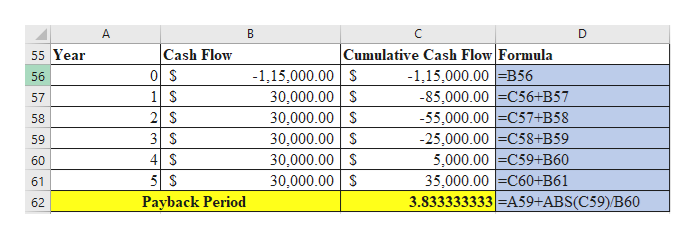# The management of Digital Waves, Inc. is considering a project with a net cost of\$115,000 and an annual net cash inflow estimated at \$30,000 over the project's life of 5years. The company has a cost of capital of 6 percent. The project under considerationhas risk that is typical for the company.a. What is the project's payback period?b. What is the project's NPV?c. What is the project's IRR?d. What is the project’s PI?

Question
2 views

The management of Digital Waves, Inc. is considering a project with a net cost of
\$115,000 and an annual net cash inflow estimated at \$30,000 over the project's life of 5
years. The company has a cost of capital of 6 percent. The project under consideration
has risk that is typical for the company.
a. What is the project's payback period?
b. What is the project's NPV?
c. What is the project's IRR?
d. What is the project’s PI?

check_circle

Step 1

a) Payback period is the number of years it would take for the project’s expected cash in flows to recover the investment cost.

It is calculated as below:

Here,

A = Last period or year in which the cumulative cash flow is negative

B = absolute vale of the cumulative cash flow at the period A

C = total cash inflow just after the period A

Step 2

Excel working for the payback period of the project.help_outlineImage TranscriptioncloseCash Flow Cumulative Cash Flow Formula -1,15,000.00 =B56 -85,000.00 =C56+B57 -55,000.00 =C57+B58 -25,000.00 =C58+B59 5,000.00 =C59+B60 35,000.00 =C60+B61 3.833333333 =A59+ABS(C59)/B60 55 Year -1,15,000.00 S 30,000.00 S 30,000.00 S 30,000.00 S 30,000.00 S 30,000.00 S 56 1 \$ 2 S 3 S 4 S 57 58 59 60 61 Payback Period 62 fullscreen
Step 3

### Want to see the full answer?

See Solution

#### Want to see this answer and more?

Solutions are written by subject experts who are available 24/7. Questions are typically answered within 1 hour.*

See Solution
*Response times may vary by subject and question.
Tagged in